Python Modules / NumPy Tutorial -# NumPy Tutorial

NumPy is a Python library.

NumPy is used for working with arrays.

NumPy is short for “Numerical Python”.

Starting with a basic introduction and ends up with creating and plotting random data sets, and working with NumPy functions:

## What is NumPy?

NumPy is a Python library used for working with arrays.

It also has functions for working in domain of linear algebra, fourier transform, and matrices.

NumPy was created in 2005 by Travis Oliphant. It is an open source project and you can use it freely.

NumPy stands for Numerical Python.

## Why Use NumPy?

In Python we have lists that serve the purpose of arrays, but they are slow to process.

NumPy aims to provide an array object that is up to 50x faster than traditional Python lists.

The array object in NumPy is called `ndarray`, it provides a lot of supporting functions that make working with `ndarray` very easy.

Arrays are very frequently used in data science, where speed and resources are very important.

Data Science: is a branch of computer science where we study how to store, use and analyze data for deriving information from it.

## Why is NumPy Faster Than Lists?

NumPy arrays are stored at one continuous place in memory unlike lists, so processes can access and manipulate them very efficiently.

This behavior is called locality of reference in computer science.

This is the main reason why NumPy is faster than lists. Also it is optimized to work with latest CPU architectures.

## Which Language is NumPy written in?

NumPy is a Python library and is written partially in Python, but most of the parts that require fast computation are written in C or C++.

## Where is the NumPy Codebase?

The source code for NumPy is located at this github repository https://github.com/numpy/numpy

github: enables many people to work on the same codebase.

# NumPy Getting Started

## Installation of NumPy

If you have Python and PIP already installed on a system, then installation of NumPy is very easy.

Install it using this command:

If this command fails, then use a python distribution that already has NumPy installed like, Anaconda, Spyder etc.

## Import NumPy

Once NumPy is installed, import it in your applications by adding the `import` keyword:

import numpy

Now NumPy is imported and ready to use.

### Example

`import numpyarr = numpy.array([1, 2, 3, 4, 5])print(arr)`

Try it Yourself »

## NumPy as np

NumPy is usually imported under the `np` alias.

alias: In Python alias are an alternate name for referring to the same thing.

Create an alias with the `as` keyword while importing:

import numpy as np

Now the NumPy package can be referred to as `np` instead of `numpy`.

### Example

`import numpy as nparr = np.array([1, 2, 3, 4, 5])print(arr)`

Try it Yourself »

## Checking NumPy Version

The version string is stored under `__version__` attribute.

### Example

`import numpy as npprint(np.__version__)`

Try it Yourself »

# NumPy Creating Arrays

## Create a NumPy ndarray Object

NumPy is used to work with arrays. The array object in NumPy is called `ndarray`.

We can create a NumPy `ndarray` object by using the `array()` function.

### Example

`import numpy as nparr = np.array([1, 2, 3, 4, 5])print(arr)print(type(arr))`

Try it Yourself »

type(): This built-in Python function tells us the type of the object passed to it. Like in above code it shows that `arr` is `numpy.ndarray` type.

To create an `ndarray`, we can pass a list, tuple or any array-like object into the `array()` method, and it will be converted into an `ndarray`:

### Example

Use a tuple to create a NumPy array:

`import numpy as nparr = np.array((1, 2, 3, 4, 5))print(arr)`

Try it Yourself »

## Dimensions in Arrays

A dimension in arrays is one level of array depth (nested arrays).

nested array: are arrays that have arrays as their elements.

## 0-D Arrays

0-D arrays, or Scalars, are the elements in an array. Each value in an array is a 0-D array.

### Example

Create a 0-D array with value 42

`import numpy as nparr = np.array(42)print(arr)`

Try it Yourself »

## 1-D Arrays

An array that has 0-D arrays as its elements is called uni-dimensional or 1-D array.

These are the most common and basic arrays.

### Example

Create a 1-D array containing the values 1,2,3,4,5:

`import numpy as nparr = np.array([1, 2, 3, 4, 5])print(arr)`

Try it Yourself »

## 2-D Arrays

An array that has 1-D arrays as its elements is called a 2-D array.

These are often used to represent matrix or 2nd order tensors.

NumPy has a whole sub module dedicated towards matrix operations called `numpy.mat`

### Example

Create a 2-D array containing two arrays with the values 1,2,3 and 4,5,6:

`import numpy as nparr = np.array([[1, 2, 3], [4, 5, 6]])print(arr)`

Try it Yourself »

## 3-D arrays

An array that has 2-D arrays (matrices) as its elements is called 3-D array.

These are often used to represent a 3rd order tensor.

### Example

Create a 3-D array with two 2-D arrays, both containing two arrays with the values 1,2,3 and 4,5,6:

`import numpy as nparr = np.array([[[1, 2, 3], [4, 5, 6]], [[1, 2, 3], [4, 5, 6]]])print(arr)`

Try it Yourself »

## Check Number of Dimensions?

NumPy Arrays provides the `ndim` attribute that returns an integer that tells us how many dimensions the array have.

### Example

Check how many dimensions the arrays have:

`import numpy as npa = np.array(42)b = np.array([1, 2, 3, 4, 5])c = np.array([[1, 2, 3], [4, 5, 6]])d = np.array([[[1, 2, 3], [4, 5, 6]], [[1, 2, 3], [4, 5, 6]]])print(a.ndim)print(b.ndim)print(c.ndim)print(d.ndim)`

Try it Yourself »

## Higher Dimensional Arrays

An array can have any number of dimensions.

When the array is created, you can define the number of dimensions by using the `ndmin` argument.

### Example

Create an array with 5 dimensions and verify that it has 5 dimensions:

`import numpy as nparr = np.array([1, 2, 3, 4], ndmin=5)`

print(arr)
print(‘number of dimensions :’, arr.ndim)

Try it Yourself »

In this array the innermost dimension (5th dim) has 4 elements, the 4th dim has 1 element that is the vector, the 3rd dim has 1 element that is the matrix with the vector, the 2nd dim has 1 element that is 3D array and 1st dim has 1 element that is a 4D array.

## Exercise:

Insert the correct method for creating a NumPy array.

```arr = np.([1, 2, 3, 4, 5])
```

Start the Exercise

# NumPy Array Indexing

## Access Array Elements

Array indexing is the same as accessing an array element.

You can access an array element by referring to its index number.

The indexes in NumPy arrays start with 0, meaning that the first element has index 0, and the second has index 1 etc.

### Example

Get the first element from the following array:

`import numpy as nparr = np.array([1, 2, 3, 4])print(arr)`

Try it Yourself »

### Example

Get the second element from the following array.

`import numpy as nparr = np.array([1, 2, 3, 4])`

print(arr)

Try it Yourself »

### Example

Get third and fourth elements from the following array and add them.

`import numpy as nparr = np.array([1, 2, 3, 4])print(arr + arr)`

Try it Yourself »

## Access 2-D Arrays

To access elements from 2-D arrays we can use comma separated integers representing the dimension and the index of the element.

Think of 2-D arrays like a table with rows and columns, where the row represents the dimension and the index represents the column.

### Example

Access the element on the first row, second column:

`import numpy as nparr = np.array([[1,2,3,4,5], [6,7,8,9,10]])print('2nd element on 1st row: ', arr[0, 1])`

Try it Yourself »

### Example

Access the element on the 2nd row, 5th column:

`import numpy as nparr = np.array([[1,2,3,4,5], [6,7,8,9,10]])print('5th element on 2nd row: ', arr[1, 4])`

Try it Yourself »

## Access 3-D Arrays

To access elements from 3-D arrays we can use comma separated integers representing the dimensions and the index of the element.

### Example

Access the third element of the second array of the first array:

`import numpy as nparr = np.array([[[1, 2, 3], [4, 5, 6]], [[7, 8, 9], [10, 11, 12]]])print(arr[0, 1, 2])`

Try it Yourself »

### Example Explained

`arr[0, 1, 2]` prints the value `6`.

And this is why:

The first number represents the first dimension, which contains two arrays:
[[1, 2, 3], [4, 5, 6]]
and:
[[7, 8, 9], [10, 11, 12]]
Since we selected `0`, we are left with the first array:
[[1, 2, 3], [4, 5, 6]]

The second number represents the second dimension, which also contains two arrays:
[1, 2, 3]
and:
[4, 5, 6]
Since we selected `1`, we are left with the second array:
[4, 5, 6]

The third number represents the third dimension, which contains three values:
4
5
6
Since we selected `2`, we end up with the third value:
6

## Negative Indexing

Use negative indexing to access an array from the end.

### Example

Print the last element from the 2nd dim:

`import numpy as nparr = np.array([[1,2,3,4,5], [6,7,8,9,10]])print('Last element from 2nd dim: ', arr[1, -1])`

Try it Yourself »

## Exercise:

Insert the correct syntax for printing the first item in the array.

```arr = np.array([1, 2, 3, 4, 5])

print(arr)
```

Start the Exercise

# NumPy Array Slicing

## Slicing arrays

Slicing in python means taking elements from one given index to another given index.

We pass slice instead of index like this: `[start:end]`.

We can also define the step, like this: `[start:end:step]`.

If we don’t pass start its considered 0

If we don’t pass end its considered length of array in that dimension

If we don’t pass step its considered 1

### Example

Slice elements from index 1 to index 5 from the following array:

`import numpy as nparr = np.array([1, 2, 3, 4, 5, 6, 7])print(arr[1:5])`

Try it Yourself »

Note: The result includes the start index, but excludes the end index.

### Example

Slice elements from index 4 to the end of the array:

`import numpy as nparr = np.array([1, 2, 3, 4, 5, 6, 7])print(arr[4:])`

Try it Yourself »

### Example

Slice elements from the beginning to index 4 (not included):

`import numpy as nparr = np.array([1, 2, 3, 4, 5, 6, 7])print(arr[:4])`

Try it Yourself »

## Negative Slicing

Use the minus operator to refer to an index from the end:

### Example

Slice from the index 3 from the end to index 1 from the end:

`import numpy as nparr = np.array([1, 2, 3, 4, 5, 6, 7])print(arr[-3:-1])`

Try it Yourself »

## STEP

Use the `step` value to determine the step of the slicing:

### Example

Return every other element from index 1 to index 5:

`import numpy as nparr = np.array([1, 2, 3, 4, 5, 6, 7])print(arr[1:5:2])`

Try it Yourself »

### Example

Return every other element from the entire array:

`import numpy as nparr = np.array([1, 2, 3, 4, 5, 6, 7])print(arr[::2])`

Try it Yourself »

## Slicing 2-D Arrays

### Example

From the second element, slice elements from index 1 to index 4 (not included):

`import numpy as nparr = np.array([[1, 2, 3, 4, 5], [6, 7, 8, 9, 10]])print(arr[1, 1:4])`

Try it Yourself »

Note: Remember that second element has index 1.

### Example

From both elements, return index 2:

`import numpy as nparr = np.array([[1, 2, 3, 4, 5], [6, 7, 8, 9, 10]])print(arr[0:2, 2])`

Try it Yourself »

### Example

From both elements, slice index 1 to index 4 (not included), this will return a 2-D array:

`import numpy as nparr = np.array([[1, 2, 3, 4, 5], [6, 7, 8, 9, 10]])print(arr[0:2, 1:4])`

Try it Yourself »

## Exercise:

Insert the correct slicing syntax to print the following selection of the array:

Everything from (including) the second item to (not including) the fifth item.

```arr = np.array([10, 15, 20, 25, 30, 35, 40])

print(arr)
```

Start the Exercise

# NumPy Data Types

## Data Types in Python

By default Python have these data types:

• `strings` – used to represent text data, the text is given under quote marks. e.g. “ABCD”
• `integer` – used to represent integer numbers. e.g. -1, -2, -3
• `float` – used to represent real numbers. e.g. 1.2, 42.42
• `boolean` – used to represent True or False.
• `complex` – used to represent complex numbers. e.g. 1.0 + 2.0j, 1.5 + 2.5j

## Data Types in NumPy

NumPy has some extra data types, and refer to data types with one character, like `i` for integers, `u` for unsigned integers etc.

Below is a list of all data types in NumPy and the characters used to represent them.

• `i` – integer
• `b` – boolean
• `u` – unsigned integer
• `f` – float
• `c` – complex float
• `m` – timedelta
• `M` – datetime
• `O` – object
• `S` – string
• `U` – unicode string
• `V` – fixed chunk of memory for other type ( void )

## Checking the Data Type of an Array

The NumPy array object has a property called `dtype` that returns the data type of the array:

### Example

Get the data type of an array object:

`import numpy as nparr = np.array([1, 2, 3, 4])print(arr.dtype)`

Try it Yourself »

### Example

Get the data type of an array containing strings:

`import numpy as nparr = np.array(['apple', 'banana', 'cherry'])print(arr.dtype)`

Try it Yourself »

## Creating Arrays With a Defined Data Type

We use the `array()` function to create arrays, this function can take an optional argument: `dtype` that allows us to define the expected data type of the array elements:

### Example

Create an array with data type string:

`import numpy as nparr = np.array([1, 2, 3, 4], dtype='S')print(arr)print(arr.dtype)`

Try it Yourself »

For `i``u``f``S` and `U` we can define size as well.

### Example

Create an array with data type 4 bytes integer:

`import numpy as nparr = np.array([1, 2, 3, 4], dtype='i4')print(arr)print(arr.dtype)`

Try it Yourself »

## What if a Value Can Not Be Converted?

If a type is given in which elements can’t be casted then NumPy will raise a ValueError.

ValueError: In Python ValueError is raised when the type of passed argument to a function is unexpected/incorrect.

### Example

A non integer string like ‘a’ can not be converted to integer (will raise an error):

`import numpy as nparr = np.array(['a', '2', '3'], dtype='i')`

Try it Yourself »

## Converting Data Type on Existing Arrays

The best way to change the data type of an existing array, is to make a copy of the array with the `astype()` method.

The `astype()` function creates a copy of the array, and allows you to specify the data type as a parameter.

The data type can be specified using a string, like `'f'` for float, `'i'` for integer etc. or you can use the data type directly like `float` for float and `int` for integer.

### Example

Change data type from float to integer by using `'i'` as parameter value:

`import numpy as nparr = np.array([1.1, 2.1, 3.1])newarr = arr.astype('i')print(newarr)print(newarr.dtype)`

Try it Yourself »

### Example

Change data type from float to integer by using `int` as parameter value:

`import numpy as nparr = np.array([1.1, 2.1, 3.1])newarr = arr.astype(int)print(newarr)print(newarr.dtype)`

Try it Yourself »

### Example

Change data type from integer to boolean:

`import numpy as nparr = np.array([1, 0, 3])newarr = arr.astype(bool)print(newarr)print(newarr.dtype)`

Try it Yourself »

## Exercise:

NumPy uses a character to represent each of the following data types, which one?

```i = integer
= boolean
= unsigned integer
= float
= complex float
= timedelta
= datetime
= object
= string
```

Start the Exercise

# NumPy Array Copy vs View

## The Difference Between Copy and View

The main difference between a copy and a view of an array is that the copy is a new array, and the view is just a view of the original array.

The copy owns the data and any changes made to the copy will not affect original array, and any changes made to the original array will not affect the copy.

The view does not own the data and any changes made to the view will affect the original array, and any changes made to the original array will affect the view.

## COPY:

### Example

Make a copy, change the original array, and display both arrays:

`import numpy as nparr = np.array([1, 2, 3, 4, 5])x = arr.copy()arr = 42print(arr)print(x)`

Try it Yourself »

The copy SHOULD NOT be affected by the changes made to the original array.

## VIEW:

### Example

Make a view, change the original array, and display both arrays:

`import numpy as nparr = np.array([1, 2, 3, 4, 5])x = arr.view()arr = 42print(arr)print(x)`

Try it Yourself »

The view SHOULD be affected by the changes made to the original array.

### Example

Make a view, change the view, and display both arrays:

`import numpy as nparr = np.array([1, 2, 3, 4, 5])x = arr.view()x = 31print(arr)print(x)`

Try it Yourself »

The original array SHOULD be affected by the changes made to the view.

## Check if Array Owns its Data

As mentioned above, copies owns the data, and views does not own the data, but how can we check this?

Every NumPy array has the attribute `base` that returns `None` if the array owns the data.

Otherwise, the `base`  attribute refers to the original object.

### Example

Print the value of the base attribute to check if an array owns it’s data or not:

`import numpy as nparr = np.array([1, 2, 3, 4, 5])x = arr.copy()y = arr.view()print(x.base)print(y.base)`

Try it Yourself »

The copy returns `None`.
The view returns the original array.

## Exercise:

Use the correct method to make a copy of the array.

```arr = np.array([1, 2, 3, 4, 5])

x = arr.
```

Start the Exercise

# NumPy Array Shape

## Shape of an Array

The shape of an array is the number of elements in each dimension.

## Get the Shape of an Array

NumPy arrays have an attribute called `shape` that returns a tuple with each index having the number of corresponding elements.

### Example

Print the shape of a 2-D array:

import numpy as np

arr = np.array([, ])

print(arr.shape)
Try it Yourself »

The example above returns `(2, 4)`, which means that the array has 2 dimensions, where the first dimension has 2 elements and the second has 4.

### Example

Create an array with 5 dimensions using `ndmin` using a vector with values 1,2,3,4 and verify that last dimension has value 4:

import numpy as np

arr = np.array(, ndmin=5)

print(arr)
print(‘shape of array :’, arr.shape)
Try it Yourself »

## What does the shape tuple represent?

Integers at every index tells about the number of elements the corresponding dimension has.

In the example above at index-4 we have value 4, so we can say that 5th ( 4 + 1 th) dimension has 4 elements.

## Exercise:

Use the correct NumPy syntax to check the shape of an array.

```arr = np.array([1, 2, 3, 4, 5])

print(arr.)
```

Start the Exercise

# NumPy Array Reshaping

## Reshaping arrays

Reshaping means changing the shape of an array.

The shape of an array is the number of elements in each dimension.

By reshaping we can add or remove dimensions or change number of elements in each dimension.

## Reshape From 1-D to 2-D

### Example

Convert the following 1-D array with 12 elements into a 2-D array.

The outermost dimension will have 4 arrays, each with 3 elements:

import numpy as np

arr = np.array()

newarr = arr.reshape(43)

print(newarr)
Try it Yourself »

## Reshape From 1-D to 3-D

### Example

Convert the following 1-D array with 12 elements into a 3-D array.

The outermost dimension will have 2 arrays that contains 3 arrays, each with 2 elements:

import numpy as np

arr = np.array()

newarr = arr.reshape(232)

print(newarr)
Try it Yourself »

## Can We Reshape Into any Shape?

Yes, as long as the elements required for reshaping are equal in both shapes.

We can reshape an 8 elements 1D array into 4 elements in 2 rows 2D array but we cannot reshape it into a 3 elements 3 rows 2D array as that would require 3×3 = 9 elements.

### Example

Try converting 1D array with 8 elements to a 2D array with 3 elements in each dimension (will raise an error):

import numpy as np

arr = np.array()

newarr = arr.reshape(33)

print(newarr)
Try it Yourself »

## Returns Copy or View?

### Example

Check if the returned array is a copy or a view:

import numpy as np

arr = np.array()

print(arr.reshape(24).base)
Try it Yourself »

The example above returns the original array, so it is a view.

## Unknown Dimension

You are allowed to have one “unknown” dimension.

Meaning that you do not have to specify an exact number for one of the dimensions in the reshape method.

Pass `-1` as the value, and NumPy will calculate this number for you.

### Example

Convert 1D array with 8 elements to 3D array with 2×2 elements:

import numpy as np

arr = np.array()

newarr = arr.reshape(22, –1)

print(newarr)
Try it Yourself »

Note: We can not pass `-1` to more than one dimension.

## Flattening the arrays

Flattening array means converting a multidimensional array into a 1D array.

We can use `reshape(-1)` to do this.

### Example

Convert the array into a 1D array:

import numpy as np

arr = np.array([, ])

newarr = arr.reshape(-1)

print(newarr)
Try it Yourself »

Note: There are a lot of functions for changing the shapes of arrays in numpy `flatten``ravel` and also for rearranging the elements `rot90``flip``fliplr``flipud` etc. These fall under Intermediate to Advanced section of numpy.

## Exercise:

Use the correct NumPy method to change the shape of an array from 1-D to 2-D.

```arr = np.array([1, 2, 3, 4, 5, 6, 7, 8, 9, 10, 11, 12])

newarr = arr.(4, 3)
```

Start the Exercise

## Iterating Arrays

Iterating means going through elements one by one.

As we deal with multi-dimensional arrays in numpy, we can do this using basic `for` loop of python.

If we iterate on a 1-D array it will go through each element one by one.

### Example

Iterate on the elements of the following 1-D array:

import numpy as np

arr = np.array()

for x in arr:
print(x)
Try it Yourself »

## Iterating 2-D Arrays

In a 2-D array it will go through all the rows.

### Example

Iterate on the elements of the following 2-D array:

import numpy as np

arr = np.array([, ])

for x in arr:
print(x)
Try it Yourself »

If we iterate on a n-D array it will go through n-1th dimension one by one.

To return the actual values, the scalars, we have to iterate the arrays in each dimension.

### Example

Iterate on each scalar element of the 2-D array:

import numpy as np

arr = np.array([, ])

for x in arr:
for y in x:
print(y)
Try it Yourself »

## Iterating 3-D Arrays

In a 3-D array it will go through all the 2-D arrays.

### Example

Iterate on the elements of the following 3-D array:

import numpy as np

arr = np.array([[, ], [, ]])

for x in arr:
print(x)
Try it Yourself »

To return the actual values, the scalars, we have to iterate the arrays in each dimension.

### Example

Iterate down to the scalars:

import numpy as np

arr = np.array([[, ], [, ]])

for x in arr:
for y in x:
for z in y:
print(z)
Try it Yourself »

## Iterating Arrays Using nditer()

The function `nditer()` is a helping function that can be used from very basic to very advanced iterations. It solves some basic issues which we face in iteration, lets go through it with examples.

### Iterating on Each Scalar Element

In basic `for` loops, iterating through each scalar of an array we need to use n `for` loops which can be difficult to write for arrays with very high dimensionality.

### Example

Iterate through the following 3-D array:

import numpy as np

arr = np.array([[, ], [, ]])

for x in np.nditer(arr):
print(x)
Try it Yourself »

## Iterating Array With Different Data Types

We can use `op_dtypes` argument and pass it the expected datatype to change the datatype of elements while iterating.

NumPy does not change the data type of the element in-place (where the element is in array) so it needs some other space to perform this action, that extra space is called buffer, and in order to enable it in `nditer()` we pass `flags=['buffered']`.

### Example

Iterate through the array as a string:

import numpy as np

arr = np.array()

for x in np.nditer(arr, flags=[‘buffered’], op_dtypes=[‘S’]):
print(x)
Try it Yourself »

## Iterating With Different Step Size

We can use filtering and followed by iteration.

### Example

Iterate through every scalar element of the 2D array skipping 1 element:

import numpy as np

arr = np.array([, ])

for x in np.nditer(arr[:, ::2]):
print(x)
Try it Yourself »

## Enumerated Iteration Using ndenumerate()

Enumeration means mentioning sequence number of somethings one by one.

Sometimes we require corresponding index of the element while iterating, the `ndenumerate()` method can be used for those usecases.

### Example

Enumerate on following 1D arrays elements:

import numpy as np

arr = np.array()

for idx, x in np.ndenumerate(arr):
print(idx, x)
Try it Yourself »

### Example

Enumerate on following 2D array’s elements:

import numpy as np

arr = np.array([, ])

for idx, x in np.ndenumerate(arr):
print(idx, x)
Try it Yourself »

# NumPy Joining Array

## Joining NumPy Arrays

Joining means putting contents of two or more arrays in a single array.

In SQL we join tables based on a key, whereas in NumPy we join arrays by axes.

We pass a sequence of arrays that we want to join to the `concatenate()` function, along with the axis. If axis is not explicitly passed, it is taken as 0.

### Example

Join two arrays

import numpy as np

arr1 = np.array()

arr2 = np.array()

arr = np.concatenate((arr1, arr2))

print(arr)
Try it Yourself »

### Example

Join two 2-D arrays along rows (axis=1):

import numpy as np

arr1 = np.array([, ])

arr2 = np.array([, ])

arr = np.concatenate((arr1, arr2), axis=1)

print(arr)
Try it Yourself »

## Joining Arrays Using Stack Functions

Stacking is same as concatenation, the only difference is that stacking is done along a new axis.

We can concatenate two 1-D arrays along the second axis which would result in putting them one over the other, ie. stacking.

We pass a sequence of arrays that we want to join to the `stack()` method along with the axis. If axis is not explicitly passed it is taken as 0.

### Example

import numpy as np

arr1 = np.array()

arr2 = np.array()

arr = np.stack((arr1, arr2), axis=1)

print(arr)
Try it Yourself »

## Stacking Along Rows

NumPy provides a helper function: `hstack()` to stack along rows.

### Example

import numpy as np

arr1 = np.array()

arr2 = np.array()

arr = np.hstack((arr1, arr2))

print(arr)
Try it Yourself »

## Stacking Along Columns

NumPy provides a helper function: `vstack() ` to stack along columns.

### Example

import numpy as np

arr1 = np.array()

arr2 = np.array()

arr = np.vstack((arr1, arr2))

print(arr)
Try it Yourself »

## Stacking Along Height (depth)

NumPy provides a helper function: `dstack()` to stack along height, which is the same as depth.

### Example

import numpy as np

arr1 = np.array()

arr2 = np.array()

arr = np.dstack((arr1, arr2))

print(arr)
Try it Yourself »

## Exercise:

Use a correct NumPy method to join two arrays into a single array.

```arr1 = np.array([1, 2, 3])

arr2 = np.array([4, 5, 6])

arr = np.((arr1, arr2))
```

Start the Exercise

# NumPy Splitting Array

## Splitting NumPy Arrays

Splitting is reverse operation of Joining.

Joining merges multiple arrays into one and Splitting breaks one array into multiple.

We use `array_split()` for splitting arrays, we p

## Searching Arrays

You can search an array for a certain value, and return the indexes that get a match.

To search an array, use the `where()` method.

### Example

Find the indexes where the value is 4:

import numpy as np

arr = np.array()

x = np.where(arr == 4)

print(x)
Try it Yourself »

The example above will return a tuple: `(array([3, 5, 6],)`

Which means that the value 4 is present at index 3, 5, and 6.

### Example

Find the indexes where the values are even:

import numpy as np

arr = np.array()

x = np.where(arr%2 == 0)

print(x)
Try it Yourself »

### Example

Find the indexes where the values are odd:

import numpy as np

arr = np.array()

x = np.where(arr%2 == 1)

print(x)
Try it Yourself »

## Search Sorted

There is a method called `searchsorted()` which performs a binary search in the array, and returns the index where the specified value would be inserted to maintain the search order.

The `searchsorted()` method is assumed to be used on sorted arrays.

### Example

Find the indexes where the value 7 should be inserted:

import numpy as np

arr = np.array()

x = np.searchsorted(arr, 7)

print(x)
Try it Yourself »

Example explained: The number 7 should be inserted on index 1 to remain the sort order.

The method starts the search from the left and returns the first index where the number 7 is no longer larger than the next value.

### Search From the Right Side

By default the left most index is returned, but we can give `side='right'` to return the right most index instead.

### Example

Find the indexes where the value 7 should be inserted, starting from the right:

import numpy as np

arr = np.array()

x = np.searchsorted(arr, 7, side=‘right’)

print(x)
Try it Yourself »

Example explained: The number 7 should be inserted on index 2 to remain the sort order.

The method starts the search from the right and returns the first index where the number 7 is no longer less than the next value.

### Multiple Values

To search for more than one value, use an array with the specified values.

### Example

Find the indexes where the values 2, 4, and 6 should be inserted:

import numpy as np

arr = np.array()

x = np.searchsorted(arr, )

print(x)
Try it Yourself »

The return value is an array: `[1 2 3]` containing the three indexes where 2, 4, 6 would be inserted in the original array to maintain the order.

## Exercise:

Use the correct NumPy method to find all items with the value 4.

```arr = np.array([1, 2, 3, 4, 5, 4, 4])

x = np.(arr == 4)
```

Start the Exercise

# NumPy Sorting Arrays

## Sorting Arrays

Sorting means putting elements in an ordered sequence.

Ordered sequence is any sequence that has an order corresponding to elements, like numeric or alphabetical, ascending or descending.

The NumPy ndarray object has a function called `sort()`, that will sort a specified array.

### Example

Sort the array:

import numpy as np

arr = np.array()

print(np.sort(arr))
Try it Yourself »

Note: This method returns a copy of the array, leaving the original array unchanged.

You can also sort arrays of strings, or any other data type:

### Example

Sort the array alphabetically:

import numpy as np

arr = np.array([‘banana’‘cherry’‘apple’])

print(np.sort(arr))
Try it Yourself »

### Example

Sort a boolean array:

import numpy as np

arr = np.array([TrueFalseTrue])

print(np.sort(arr))
Try it Yourself »

## Sorting a 2-D Array

If you use the sort() method on a 2-D array, both arrays will be sorted:

### Example

Sort a 2-D array:

import numpy as np

arr = np.array([, ])

print(np.sort(arr))
Try it Yourself »

## Exercise:

Use the correct NumPy method to return a sorted array.

```arr = np.array([3, 2, 0, 1])

x = np.(arr)
```

Start the Exercise

# Random Numbers in NumPy

## What is a Random Number?

Random number does NOT mean a different number every time. Random means something that can not be predicted logically.

## Pseudo Random and True Random.

Computers work on programs, and programs are definitive set of instructions. So it means there must be some algorithm to generate a random number as well.

If there is a program to generate random number it can be predicted, thus it is not truly random.

Random numbers generated through a generation algorithm are called pseudo random.

Can we make truly random numbers?

Yes. In order to generate a truly random number on our computers we need to get the random data from some outside source. This outside source is generally our keystrokes, mouse movements, data on network etc.

We do not need truly random numbers, unless its related to security (e.g. encryption keys) or the basis of application is the randomness (e.g. Digital roulette wheels).

In this tutorial we will be using pseudo random numbers.

## Generate Random Number

NumPy offers the `random` module to work with random numbers.

### Example

Generate a random integer from 0 to 100:

from numpy import random

x = random.randint(100)

print(x)
Try it Yourself »

## Generate Random Float

The random module’s `rand()` method returns a random float between 0 and 1.

### Example

Generate a random float from 0 to 1:

from numpy import random

x = random.rand()

print(x)
Try it Yourself »

# Random Data Distribution

## What is Data Distribution?

Data Distribution is a list of all possible values, and how often each value occurs.

Such lists are important when working with statistics and data science.

The random module offer methods that returns randomly generated data distributions.

## Random Distribution

A random distribution is a set of random numbers that follow a certain probability density function.

Probability Density Function: A function that describes a continuous probability. i.e. probability of all values in an array.

We can generate random numbers based on defined probabilities using the `choice()` method of the `random` module.

The `choice()` method allows us to specify the probability for each value.

The probability is set by a number between 0 and 1, where 0 means that the value will never occur and 1 means that the value will always occur.

### Example

Generate a 1-D array containing 100 values, where each value has to be 3, 5, 7 or 9.

The probability for the value to be 3 is set to be 0.1

The probability for the value to be 5 is set to be 0.3

The probability for the value to be 7 is set to be 0.6

The probability for the value to be 9 is set to be 0

from numpy import random

x = random.choice(, p=[0.10.30.60.0], size=(100))

print(x)
Try it Yourself »

The sum of all probability numbers should be 1.

Even if you run the example above 100 times, the value 9 will never occur.

You can return arrays of any shape and size by specifying the shape in the `size` parameter.

### Example

Same example as above, but return a 2-D array with 3 rows, each containing 5 values.

from numpy import random

x = random.choice(, p=[0.10.30.60.0], size=(35))

print(x)

# Random Permutations

## Random Permutations of Elements

A permutation refers to an arrangement of elements. e.g. [3, 2, 1] is a permutation of [1, 2, 3] and vice-versa.

The NumPy Random module provides two methods for this: `shuffle()` and `permutation()`.

## Shuffling Arrays

Shuffle means changing arrangement of elements in-place. i.e. in the array itself.

### Example

Randomly shuffle elements of following array:

from numpy import random
import numpy as np

arr = np.array()

random.shuffle(arr)

print(arr)
Try it Yourself »

The `shuffle()` method makes changes to the original array.

## Generating Permutation of Arrays

### Example

Generate a random permutation of elements of following array:

from numpy import random
import numpy as np

arr = np.array()

print(random.permutation(arr))
Try it Yourself »

The `permutation()` method returns a re-arranged array (and leaves the original array un-changed).

# Seaborn

## Visualize Distributions With Seaborn

Seaborn is a library that uses Matplotlib underneath to plot graphs. It will be used to visualize random distributions.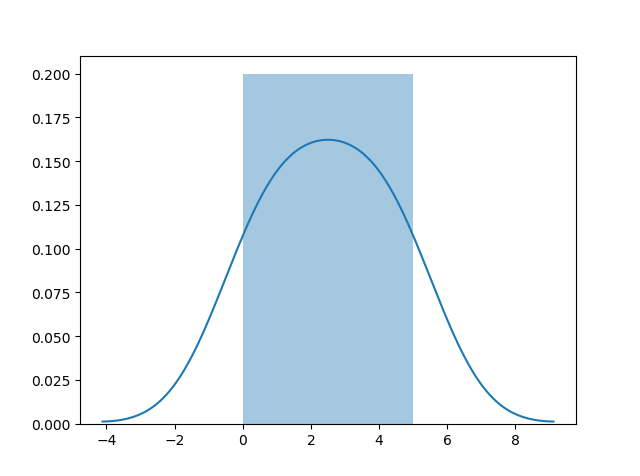## Install Seaborn.

If you have Python and PIP already installed on a system, install it using this command:

If you use Jupyter, install Seaborn using this command:

## Distplots

Distplot stands for distribution plot, it takes as input an array and plots a curve corresponding to the distribution of points in the array.

## Import Matplotlib

Import the pyplot object of the Matplotlib module in your code using the following statement:

import matplotlib.pyplot as plt

You can learn about the Matplotlib module in our Matplotlib Tutorial.

## Import Seaborn

Import the Seaborn module in your code using the following statement:

import seaborn as sns

## Plotting a Distplot

### Example

import matplotlib.pyplot as plt
import seaborn as sns

sns.distplot()

plt.show()
Try it Yourself »

## Plotting a Distplot Without the Histogram

### Example

import matplotlib.pyplot as plt
import seaborn as sns

sns.distplot(, hist=False)

plt.show()
Try it Yourself »

Note: We will be using: `sns.distplot(arr, hist=False)` to visualize random distributions in this tutorial.

# Normal (Gaussian) Distribution

## Normal Distribution

The Normal Distribution is one of the most important distributions.

It is also called the Gaussian Distribution after the German mathematician Carl Friedrich Gauss.

It fits the probability distribution of many events, eg. IQ Scores, Heartbeat etc.

Use the `random.normal()` method to get a Normal Data Distribution.

It has three parameters:

`loc` – (Mean) where the peak of the bell exists.

`scale` – (Standard Deviation) how flat the graph distribution should be.

`size` – The shape of the returned array.

### Example

Generate a random normal distribution of size 2×3:

from numpy import random

x = random.normal(size=(23))

print(x)
Try it Yourself »

### Example

Generate a random normal distribution of size 2×3 with mean at 1 and standard deviation of 2:

from numpy import random

x = random.normal(loc=1, scale=2, size=(23))

print(x)
Try it Yourself »

## Visualization of Normal Distribution

### Example

from numpy import random
import matplotlib.pyplot as plt
import seaborn as sns

sns.distplot(random.normal(size=1000), hist=False)

plt.show()

### Result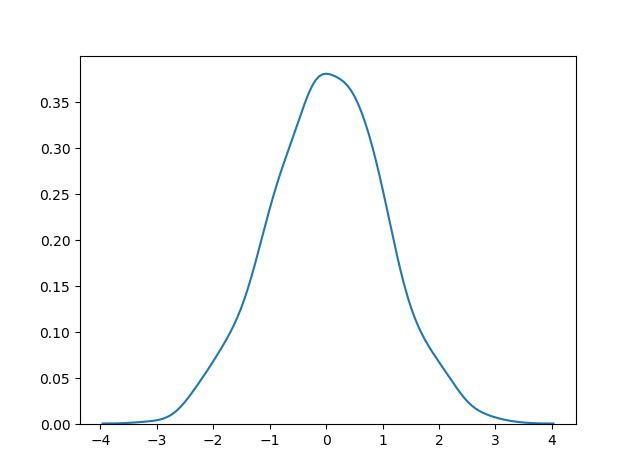Try it Yourself »

Note: The curve of a Normal Distribution is also known as the Bell Curve because of the bell-shaped curve.

# Binomial Distribution

## Binomial Distribution

Binomial Distribution is a Discrete Distribution.

It describes the outcome of binary scenarios, e.g. toss of a coin, it will either be head or tails.

It has three parameters:

`n` – number of trials.

`p` – probability of occurence of each trial (e.g. for toss of a coin 0.5 each).

`size` – The shape of the returned array.

Discrete Distribution:The distribution is defined at separate set of events, e.g. a coin toss’s result is discrete as it can be only head or tails whereas height of people is continuous as it can be 170, 170.1, 170.11 and so on.

### Example

Given 10 trials for coin toss generate 10 data points:

from numpy import random

x = random.binomial(n=10, p=0.5, size=10)

print(x)
Try it Yourself »

## Visualization of Binomial Distribution

### Example

from numpy import random
import matplotlib.pyplot as plt
import seaborn as sns

sns.distplot(random.binomial(n=10, p=0.5, size=1000), hist=True, kde=False)

plt.show()

### Result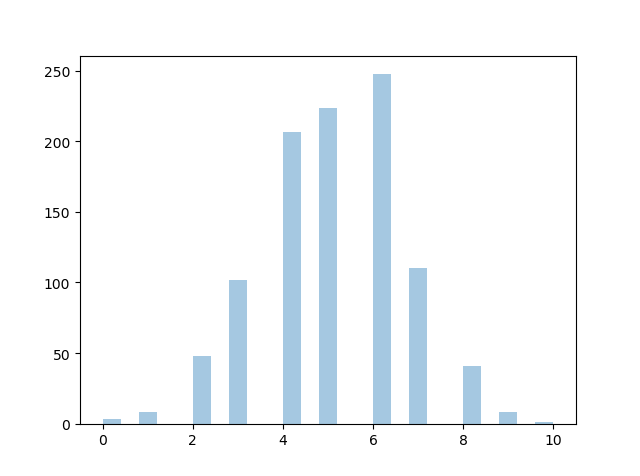Try it Yourself »

## Difference Between Normal and Binomial Distribution

The main difference is that normal distribution is continous whereas binomial is discrete, but if there are enough data points it will be quite similar to normal distribution with certain loc and scale.

### Example

from numpy import random
import matplotlib.pyplot as plt
import seaborn as sns

sns.distplot(random.normal(loc=50, scale=5, size=1000), hist=False, label=‘normal’)
sns.distplot(random.binomial(n=100, p=0.5, size=1000), hist=False, label=‘binomial’)

plt.show()

### Result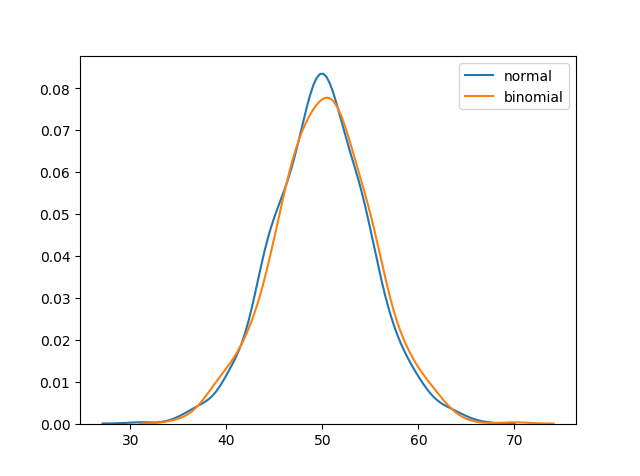Try it Yourself »

# Poisson Distribution

## Poisson Distribution

Poisson Distribution is a Discrete Distribution.

It estimates how many times an event can happen in a specified time. e.g. If someone eats twice a day what is probability he will eat thrice?

It has two parameters:

`lam` – rate or known number of occurences e.g. 2 for above problem.

`size` – The shape of the returned array.

### Example

Generate a random 1×10 distribution for occurence 2:

from numpy import random

x = random.poisson(lam=2, size=10)

print(x)
Try it Yourself »

## Visualization of Poisson Distribution

### Example

from numpy import random
import matplotlib.pyplot as plt
import seaborn as sns

sns.distplot(random.poisson(lam=2, size=1000), kde=False)

plt.show()

### Result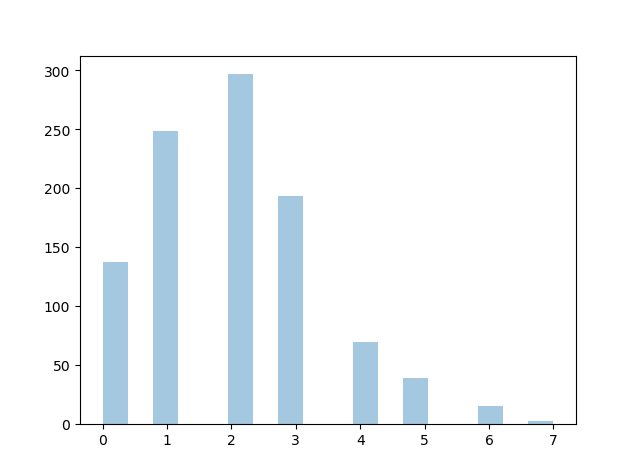Try it Yourself »

## Difference Between Normal and Poisson Distribution

Normal distribution is continous whereas poisson is discrete.

But we can see that similar to binomial for a large enough poisson distribution it will become similar to normal distribution with certain std dev and mean.

### Example

from numpy import random
import matplotlib.pyplot as plt
import seaborn as sns

sns.distplot(random.normal(loc=50, scale=7, size=1000), hist=False, label=‘normal’)
sns.distplot(random.poisson(lam=50, size=1000), hist=False, label=‘poisson’)

plt.show()

### Result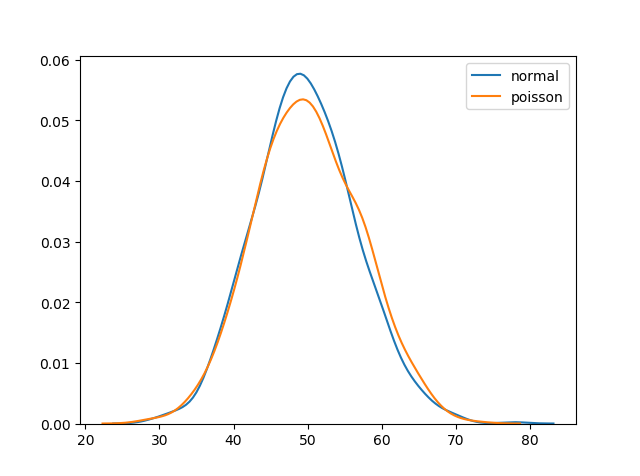Try it Yourself »

## Difference Between Poisson and Binomial Distribution

The difference is very subtle it is that, binomial distribution is for discrete trials, whereas poisson distribution is for continuous trials.

But for very large `n` and near-zero `p` binomial distribution is near identical to poisson distribution such that `n * p` is nearly equal to `lam`.

### Example

from numpy import random
import matplotlib.pyplot as plt
import seaborn as sns

sns.distplot(random.binomial(n=1000, p=0.01, size=1000), hist=False, label=‘binomial’)
sns.distplot(random.poisson(lam=10, size=1000), hist=False, label=‘poisson’)

plt.show()

### Result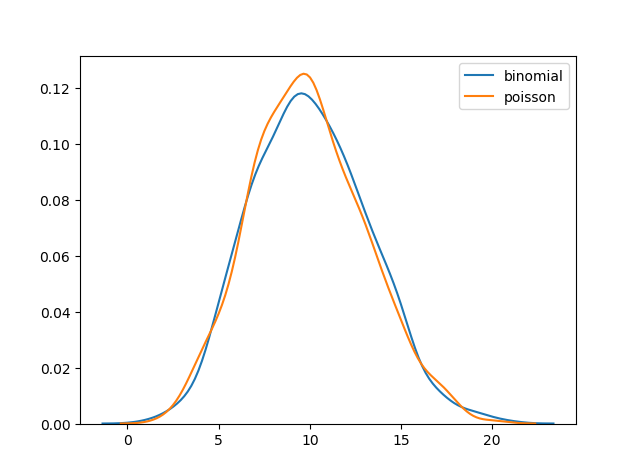Try it Yourself »

# Uniform Distribution

## Uniform Distribution

Used to describe probability where every event has equal chances of occuring.

E.g. Generation of random numbers.

It has three parameters:

`a` – lower bound – default 0 .0.

`b` – upper bound – default 1.0.

`size` – The shape of the returned array.

### Example

Create a 2×3 uniform distribution sample:

from numpy import random

x = random.uniform(size=(23))

print(x)
Try it Yourself »

## Visualization of Uniform Distribution

### Example

from numpy import random
import matplotlib.pyplot as plt
import seaborn as sns

sns.distplot(random.uniform(size=1000), hist=False)

plt.show()

### Result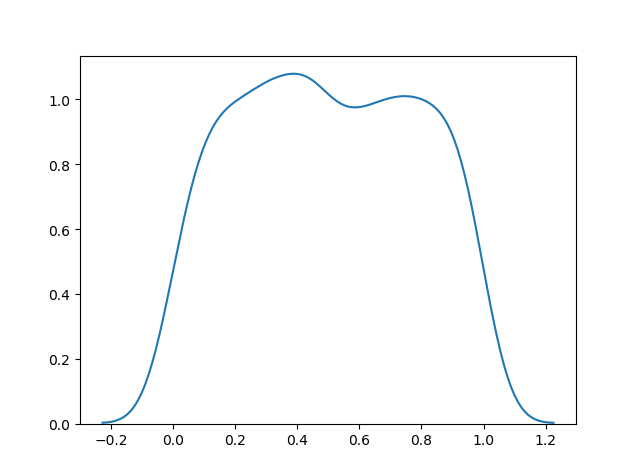Try it Yourself »

# Logistic Distribution

## Logistic Distribution

Logistic Distribution is used to describe growth.

Used extensively in machine learning in logistic regression, neural networks etc.

It has three parameters:

`loc` – mean, where the peak is. Default 0.

`scale` – standard deviation, the flatness of distribution. Default 1.

`size` – The shape of the returned array.

### Example

Draw 2×3 samples from a logistic distribution with mean at 1 and stddev 2.0:

from numpy import random

x = random.logistic(loc=1, scale=2, size=(23))

print(x)
Try it Yourself »

## Visualization of Logistic Distribution

### Example

from numpy import random
import matplotlib.pyplot as plt
import seaborn as sns

sns.distplot(random.logistic(size=1000), hist=False)

plt.show()

### Result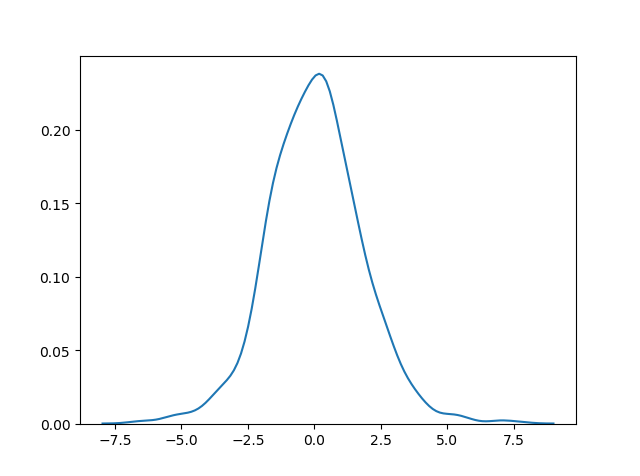Try it Yourself »

## Difference Between Logistic and Normal Distribution

Both distributions are near identical, but logistic distribution has more area under the tails. ie. It representage more possibility of occurence of an events further away from mean.

For higher value of scale (standard deviation) the normal and logistic distributions are near identical apart from the peak.

### Example

from numpy import random
import matplotlib.pyplot as plt
import seaborn as sns

sns.distplot(random.normal(scale=2, size=1000), hist=False, label=‘normal’)
sns.distplot(random.logistic(size=1000), hist=False, label=‘logistic’)

plt.show()

### Result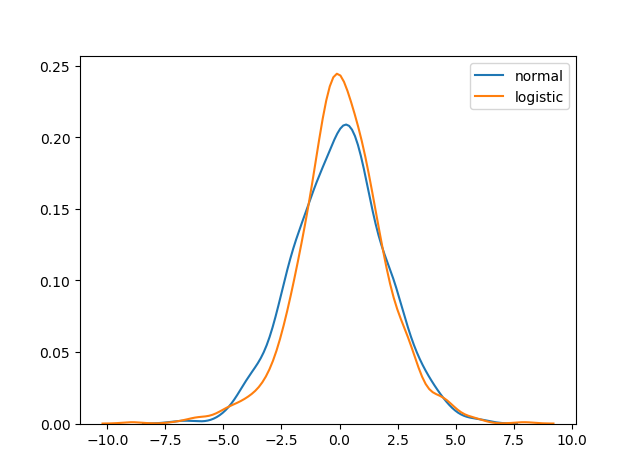Try it Yourself »

# Multinomial Distribution

## Multinomial Distribution

Multinomial distribution is a generalization of binomial distribution.

It describes outcomes of multi-nomial scenarios unlike binomial where scenarios must be only one of two. e.g. Blood type of a population, dice roll outcome.

It has three parameters:

`n` – number of possible outcomes (e.g. 6 for dice roll).

`pvals` – list of probabilties of outcomes (e.g. [1/6, 1/6, 1/6, 1/6, 1/6, 1/6] for dice roll).

`size` – The shape of the returned array.

### Example

Draw out a sample for dice roll:

from numpy import random

x = random.multinomial(n=6, pvals=[1/61/61/61/61/61/6])

print(x)
Try it Yourself »

Note: Multinomial samples will NOT produce a single value! They will produce one value for each `pval`.

Note: As they are generalization of binomial distribution their visual representation and similarity of normal distribution is same as that of multiple binomial distributions.

# Exponential Distribution

## Exponential Distribution

Exponential distribution is used for describing time till next event e.g. failure/success etc.

It has two parameters:

`scale` – inverse of rate ( see lam in poisson distribution ) defaults to 1.0.

`size` – The shape of the returned array.

### Example

Draw out a sample for exponential distribution with 2.0 scale with 2×3 size:

from numpy import random

x = random.exponential(scale=2, size=(23))

print(x)
Try it Yourself »

## Visualization of Exponential Distribution

### Example

from numpy import random
import matplotlib.pyplot as plt
import seaborn as sns

sns.distplot(random.exponential(size=1000), hist=False)

plt.show()

### Result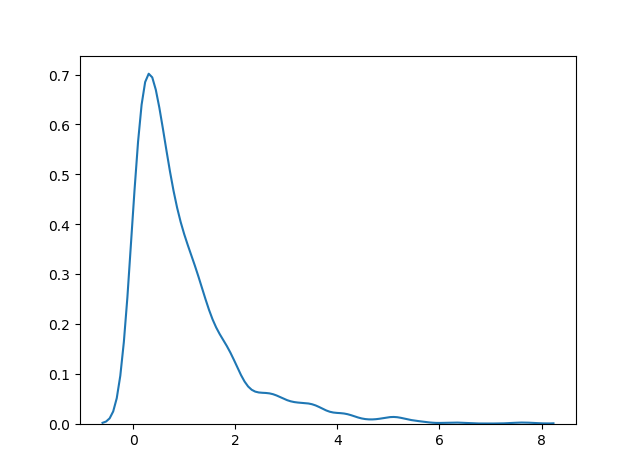Try it Yourself »

## Relation Between Poisson and Exponential Distribution

Poisson distribution deals with number of occurences of an event in a time period whereas exponential distribution deals with the time between these events.

# Chi Square Distribution

## Chi Square Distribution

Chi Square distribution is used as a basis to verify the hypothesis.

It has two parameters:

`df` – (degree of freedom).

`size` – The shape of the returned array.

### Example

Draw out a sample for chi squared distribution with degree of freedom 2 with size 2×3:

from numpy import random

x = random.chisquare(df=2, size=(23))

print(x)
Try it Yourself »

## Visualization of Chi Square Distribution

### Example

from numpy import random
import matplotlib.pyplot as plt
import seaborn as sns

sns.distplot(random.chisquare(df=1, size=1000), hist=False)

plt.show()

### Result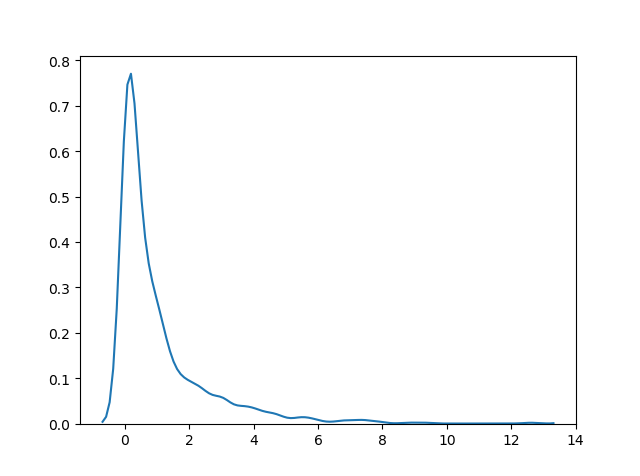Try it Yourself »

# Rayleigh Distribution

## Rayleigh Distribution

Rayleigh distribution is used in signal processing.

It has two parameters:

`scale` – (standard deviation) decides how flat the distribution will be default 1.0).

`size` – The shape of the returned array.

### Example

Draw out a sample for rayleigh distribution with scale of 2 with size 2×3:

from numpy import random

x = random.rayleigh(scale=2, size=(23))

print(x)
Try it Yourself »

## Visualization of Rayleigh Distribution

### Example

from numpy import random
import matplotlib.pyplot as plt
import seaborn as sns

sns.distplot(random.rayleigh(size=1000), hist=False)

plt.show()

### Result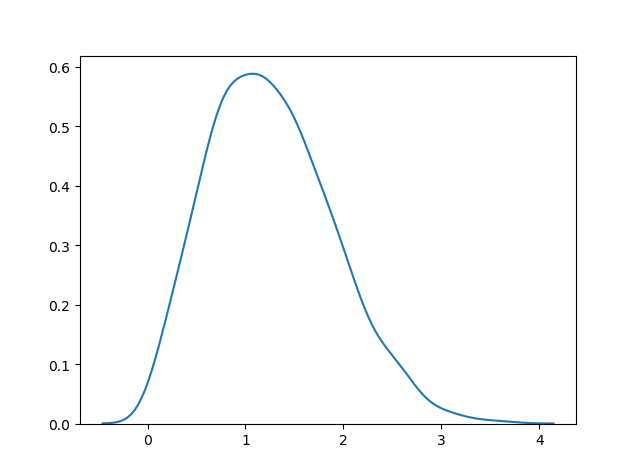Try it Yourself »

## Similarity Between Rayleigh and Chi Square Distribution

At unit stddev the and 2 degrees of freedom rayleigh and chi square represent the same distributions.

# Pareto Distribution

## areto Distribution

A distribution following Pareto’s law i.e. 80-20 distribution (20% factors cause 80% outcome).

It has two parameter:

`a` – shape parameter.

`size` – The shape of the returned array.

### Example

Draw out a sample for pareto distribution with shape of 2 with size 2×3:

from numpy import random

x = random.pareto(a=2, size=(23))

print(x)
Try it Yourself »

## Visualization of Pareto Distribution

### Example

from numpy import random
import matplotlib.pyplot as plt
import seaborn as sns

sns.distplot(random.pareto(a=2, size=1000), kde=False)

plt.show()

### Result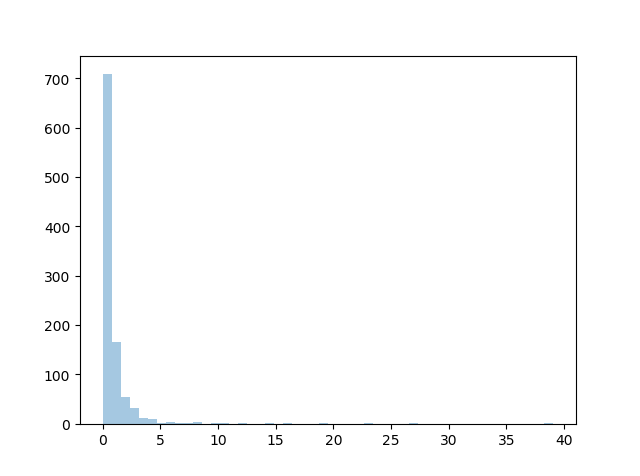# Zipf Distribution

Zipf distritutions are used to sample data based on zipf’s law.

Zipf’s Law: In a collection the nth common term is 1/n times of the most common term. E.g. 5th common word in english has occurs nearly 1/5th times as of the most used word.

It has two parameters:

`a` – distribution parameter.

`size` – The shape of the returned array.

### Example

Draw out a sample for zipf distribution with distribution parameter 2 with size 2×3:

from numpy import random

x = random.zipf(a=2, size=(23))

print(x)
Try it Yourself »

## Visualization of Zipf Distribution

Sample 1000 points but plotting only ones with value < 10 for more meaningful chart.

### Example

from numpy import random
import matplotlib.pyplot as plt
import seaborn as sns

x = random.zipf(a=2, size=1000)
sns.distplot(x[x<10], kde=False)

plt.show()

### Result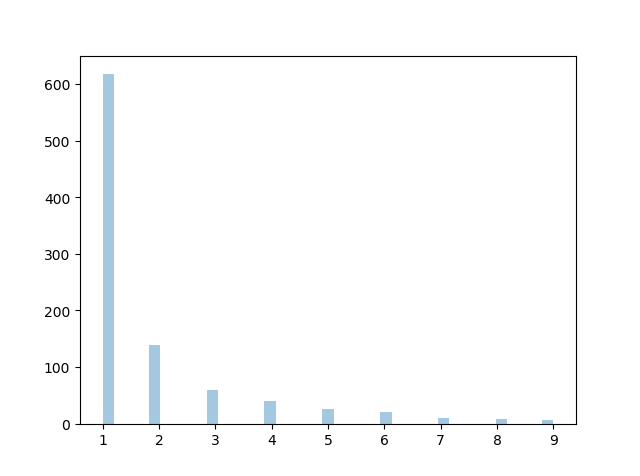Try it Yourself »

# NumPy ufuncs

## What are ufuncs?

ufuncs stands for “Universal Functions” and they are NumPy functions that operates on the `ndarray` object.

## Why use ufuncs?

ufuncs are used to implement vectorization in NumPy which is way faster than iterating over elements.

They also provide broadcasting and additional methods like reduce, accumulate etc. that are very helpful for computation.

ufuncs also take additional arguments, like:

`where` boolean array or condition defining where the operations should take place.

`dtype` defining the return type of elements.

`out` output array where the return value should be copied.

## What is Vectorization?

Converting iterative statements into a vector based operation is called vectorization.

It is faster as modern CPUs are optimized for such operations.

### Add the Elements of Two Lists

list 1: [1, 2, 3, 4]

list 2: [4, 5, 6, 7]

One way of doing it is to iterate over both of the lists and then sum each elements.

### Example

Without ufunc, we can use Python’s built-in `zip()` method:

x = 
y = 
z = []

for i, j in zip(x, y):
z.append(i + j)
print(z)
Try it Yourself »

NumPy has a ufunc for this, called `add(x, y)` that will produce the same result.

### Example

With ufunc, we can use the `add()` function:

import numpy as np

x = 
y = 

print(z)
Try it Yourself »

## How To Create Your Own ufunc

To create you own ufunc, you have to define a function, like you do with normal functions in Python, then you add it to your NumPy ufunc library with the `frompyfunc()` method.

The `frompyfunc()` method takes the following arguments:

1. `function` – the name of the function.
2. `inputs` – the number of input arguments (arrays).
3. `outputs` – the number of output arrays.

### Example

import numpy as np

return x+y

Try it Yourself »

## Check if a Function is a ufunc

Check the type of a function to check if it is a ufunc or not.

A ufunc should return `<class 'numpy.ufunc'>`.

### Example

Check if a function is a ufunc:

import numpy as np

Try it Yourself »

If it is not a ufunc, it will return another type, like this built-in NumPy function for joining two or more arrays:

### Example

Check the type of another function: concatenate():

import numpy as np

print(type(np.concatenate))
Try it Yourself »

If the function is not recognized at all, it will return an error:

### Example

Check the type of something that does not exist. This will produce an error:

import numpy as np

print(type(np.blahblah))
Try it Yourself »

To test if the function is a ufunc in an if statement, use the `numpy.ufunc` value (or `np.ufunc` if you use np as an alias for numpy):

### Example

Use an if statement to check if the function is a ufunc or not:

import numpy as np

else:
Try it Yourself »

# Simple Arithmetic

## Simple Arithmetic

You could use arithmetic operators `+` `-` `*` `/` directly between NumPy arrays, but this section discusses an extension of the same where we have functions that can take any array-like objects e.g. lists, tuples etc. and perform arithmetic conditionally.

Arithmetic Conditionally: means that we can define conditions where the arithmetic operation should happen.

All of the discussed arithmetic functions take a `where` parameter in which we can specify that condition.

The `add()` function sums the content of two arrays, and return the results in a new array.

### Example

Add the values in arr1 to the values in arr2:

import numpy as np

arr1 = np.array()
arr2 = np.array()

print(newarr)
Try it Yourself »

The example above will return [30 32 34 36 38 40] which is the sums of 10+20, 11+21, 12+22 etc.

## Subtraction

The `subtract()` function subtracts the values from one array with the values from another array, and return the results in a new array.

### Example

Subtract the values in arr2 from the values in arr1:

import numpy as np

arr1 = np.array()
arr2 = np.array()

newarr = np.subtract(arr1, arr2)

print(newarr)
Try it Yourself »

The example above will return [-10 -1 8 17 26 35] which is the result of 10-20, 20-21, 30-22 etc.

## Multiplication

The `multiply()` function multiplies the values from one array with the values from another array, and return the results in a new array.

### Example

Multiply the values in arr1 with the values in arr2:

import numpy as np

arr1 = np.array()
arr2 = np.array()

newarr = np.multiply(arr1, arr2)

print(newarr)
Try it Yourself »

The example above will return [200 420 660 920 1200 1500] which is the result of 10*20, 20*21, 30*22 etc.

## Division

The `divide()` function divides the values from one array with the values from another array, and return the results in a new array.

### Example

Divide the values in arr1 with the values in arr2:

import numpy as np

arr1 = np.array()
arr2 = np.array()

newarr = np.divide(arr1, arr2)

print(newarr)
Try it Yourself »

The example above will return [3.33333333 4. 3. 5. 25. 1.81818182] which is the result of 10/3, 20/5, 30/10 etc.

## Power

The `power()` function rises the values from the first array to the power of the values of the second array, and return the results in a new array.

### Example

Raise the valules in arr1 to the power of values in arr2:

import numpy as np

arr1 = np.array()
arr2 = np.array()

newarr = np.power(arr1, arr2)

print(newarr)
Try it Yourself »

The example above will return [1000 3200000 729000000 6553600000000 2500 0] which is the result of 10*10*10, 20*20*20*20*20, 30*30*30*30*30*30 etc.

## Remainder

Both the `mod()` and the `remainder()` functions return the remainder of the values in the first array corresponding to the values in the second array, and return the results in a new array.

### Example

Return the remainders:

import numpy as np

arr1 = np.array()
arr2 = np.array()

newarr = np.mod(arr1, arr2)

print(newarr)
Try it Yourself »

The example above will return [1 6 3 0 0 27] which is the remainders when you divide 10 with 3 (10%3), 20 with 7 (20%7) 30 with 9 (30%9) etc.

You get the same result when using the `remainder()` function:

### Example

Return the remainders:

import numpy as np

arr1 = np.array()
arr2 = np.array()

newarr = np.remainder(arr1, arr2)

print(newarr)
Try it Yourself »

## Quotient and Mod

The `divmod()` function return both the quotient and the the mod. The return value is two arrays, the first array contains the quotient and second array contains the mod.

### Example

Return the quotient and mod:

import numpy as np

arr1 = np.array()
arr2 = np.array()

newarr = np.divmod(arr1, arr2)

print(newarr)
Try it Yourself »

The example above will return:
(array([3, 2, 3, 5, 25, 1]), array([1, 6, 3, 0, 0, 27]))
The first array represents the quotients, (the integer value when you divide 10 with 3, 20 with 7, 30 with 9 etc.
The second array represents the remainders of the same divisions.

## Absolute Values

Both the `absolute()` and the `abs()` functions functions do the same absolute operation element-wise but we should use `absolute()` to avoid confusion with python’s inbuilt `math.abs()`

### Example

Return the quotient and mod:

import numpy as np

arr = np.array([-1, –2123, –4])

newarr = np.absolute(arr)

print(newarr)
Try it Yourself »

The example above will return [1 2 1 2 3 4].

# Rounding Decimals

## Rounding Decimals

There are primarily five ways of rounding off decimals in NumPy:

• truncation
• fix
• rounding
• floor
• ceil

## Truncation

Remove the decimals, and return the float number closest to zero. Use the `trunc()` and `fix()` functions.

### Example

Truncate elements of following array:

import numpy as np

arr = np.trunc([-3.16663.6667])

print(arr)
Try it Yourself »

### Example

Same example, using `fix()`:

import numpy as np

arr = np.fix([-3.16663.6667])

print(arr)
Try it Yourself »

## Rounding

The `around()` function increments preceding digit or decimal by 1 if >=5 else do nothing.

E.g. round off to 1 decimal point, 3.16666 is 3.2

### Example

Round off 3.1666 to 2 decimal places:

import numpy as np

arr = np.around(3.16662)

print(arr)
Try it Yourself »

## Floor

The floor() function rounds off decimal to nearest lower integer.

E.g. floor of 3.166 is 3.

### Example

Floor the elements of following array:

import numpy as np

arr = np.floor([-3.16663.6667])

print(arr)
Try it Yourself »

Note: The `floor()` function returns floats, unlike the `trunc()` function who returns integers.

## Ceil

The ceil() function rounds off decimal to nearest upper integer.

E.g. ceil of 3.166 is 4.

### Example

Ceil the elements of following array:

import numpy as np

arr = np.ceil([-3.16663.6667])

print(arr)
Try it Yourself »

# NumPy Logs

## Logs

NumPy provides functions to perform log at the base 2, e and 10.

We will also explore how we can take log for any base by creating a custom ufunc.

All of the log functions will place -inf or inf in the elements if the log can not be computed.

## Log at Base 2

Use the `log2()` function to perform log at the base 2.

### Example

Find log at base 2 of all elements of following array:

import numpy as np

arr = np.arange(110)

print(np.log2(arr))
Try it Yourself »

Note: The `arange(1, 10)` function returns an array with integers starting from 1 (included) to 10 (not included).

## Log at Base 10

Use the `log10()` function to perform log at the base 10.

### Example

Find log at base 10 of all elements of following array:

import numpy as np

arr = np.arange(110)

print(np.log10(arr))
Try it Yourself »

## Natural Log, or Log at Base e

Use the `log()` function to perform log at the base e.

### Example

Find log at base e of all elements of following array:

import numpy as np

arr = np.arange(110)

print(np.log(arr))
Try it Yourself »

## Log at Any Base

NumPy does not provide any function to take log at any base, so we can use the `frompyfunc()` function along with inbuilt function `math.log()` with two input parameters and one output parameter:

### Example

from math import log
import numpy as np

nplog = np.frompyfunc(log, 21)

print(nplog(10015))
Try it Yourself »

# NumPy Summations

## Summations

What is the difference between summation and addition?

Addition is done between two arguments whereas summation happens over n elements.

### Example

Add the values in arr1 to the values in arr2:

import numpy as np

arr1 = np.array()
arr2 = np.array()

print(newarr)
Try it Yourself »

Returns: `[2 4 6]`

### Example

Sum the values in arr1 and the values in arr2:

import numpy as np

arr1 = np.array()
arr2 = np.array()

newarr = np.sum([arr1, arr2])

print(newarr)
Try it Yourself »

Returns: `12`

## Summation Over an Axis

If you specify `axis=1`, NumPy will sum the numbers in each array.

### Example

Perform summation in the following array over 1st axis:

import numpy as np

arr1 = np.array()
arr2 = np.array()

newarr = np.sum([arr1, arr2], axis=1)

print(newarr)
Try it Yourself »

Returns: `[6 6]`

## Cummulative Sum

Cummulative sum means partially adding the elements in array.

E.g. The partial sum of [1, 2, 3, 4] would be [1, 1+2, 1+2+3, 1+2+3+4] = [1, 3, 6, 10].

Perfom partial sum with the `cumsum()` function.

### Example

Perform cummulative summation in the following array:

import numpy as np

arr = np.array()

newarr = np.cumsum(arr)

print(newarr)
Try it Yourself »

Returns: `[1 3 6]`

# NumPy Products

## Products

To find the product of the elements in an array, use the `prod()` function.

### Example

Find the product of the elements of this array:

import numpy as np

arr = np.array()

x = np.prod(arr)

print(x)
Try it Yourself »

Returns: `24` because 1*2*3*4 = 24

### Example

Find the product of the elements of two arrays:

import numpy as np

arr1 = np.array()
arr2 = np.array()

x = np.prod([arr1, arr2])

print(x)
Try it Yourself »

Returns: `40320` because 1*2*3*4*5*6*7*8 = 40320

## Product Over an Axis

If you specify `axis=1`, NumPy will return the product of each array.

### Example

Perform summation in the following array over 1st axis:

import numpy as np

arr1 = np.array()
arr2 = np.array()

newarr = np.prod([arr1, arr2], axis=1)

print(newarr)
Try it Yourself »

Returns: `[24 1680]`

## Cummulative Product

Cummulative product means taking the product partially.

E.g. The partial product of [1, 2, 3, 4] is [1, 1*2, 1*2*3, 1*2*3*4] = [1, 2, 6, 24]

Perfom partial sum with the `cumprod()` function.

### Example

Take cummulative product of all elements for following array:

import numpy as np

arr = np.array()

newarr = np.cumprod(arr)

print(newarr)
Try it Yourself »

Returns: `[5 30 210 1680]`

# NumPy Differences

## Differences

A discrete difference means subtracting two successive elements.

E.g. for [1, 2, 3, 4], the discrete difference would be [2-1, 3-2, 4-3] = [1, 1, 1]

To find the discrete difference, use the `diff()` function.

### Example

Compute discrete difference of the following array:

import numpy as np

arr = np.array()

newarr = np.diff(arr)

print(newarr)
Try it Yourself »

Returns: `[5 10 -20]` because 15-10=5, 25-15=10, and 5-25=-20

We can perform this operation repeatedly by giving parameter `n`.

E.g. for [1, 2, 3, 4], the discrete difference with n = 2 would be [2-1, 3-2, 4-3] = [1, 1, 1] , then, since n=2, we will do it once more, with the new result: [1-1, 1-1] = [0, 0]

### Example

Compute discrete difference of the following array twice:

import numpy as np

arr = np.array()

newarr = np.diff(arr, n=2)

print(newarr)
Try it Yourself »

Returns: `[5 -30]` because: 15-10=5, 25-15=10, and 5-25=-20 AND 10-5=5 and -20-10=-30

# NumPy LCM Lowest Common Multiple

## Finding LCM (Lowest Common Multiple)

The Lowest Common Multiple is the least number that is common multiple of both of the numbers.

### Example

Find the LCM of the following two numbers:

import numpy as np

num1 = 4
num2 = 6

x = np.lcm(num1, num2)

print(x)
Try it Yourself »

Returns: `12` because that is the lowest common multiple of both numbers (4*3=12 and 6*2=12).

## Finding LCM in Arrays

To find the Lowest Common Multiple of all values in an array, you can use the `reduce()` method.

The `reduce()` method will use the ufunc, in this case the `lcm()` function, on each element, and reduce the array by one dimension.

### Example

Find the LCM of the values of the following array:

import numpy as np

arr = np.array()

x = np.lcm.reduce(arr)

print(x)
Try it Yourself »

Returns: `18` because that is the lowest common multiple of all three numbers (3*6=18, 6*3=18 and 9*2=18).

### Example

Find the LCM of all of an array where the array contains all integers from 1 to 10:

import numpy as np

arr = np.arange(111)

x = np.lcm.reduce(arr)

print(x)
Try it Yourself »

# NumPy GCD Greatest Common Denominator

## Finding GCD (Greatest Common Denominator)

The GCD (Greatest Common Denominator), also known as HCF (Highest Common Factor) is the biggest number that is a common factor of both of the numbers.

### Example

Find the HCF of the following two numbers:

import numpy as np

num1 = 6
num2 = 9

x = np.gcd(num1, num2)

print(x)
Try it Yourself »

Returns: `3` because that is the highest number both numbers can be divided by (6/3=2 and 9/3=3).

## Finding GCD in Arrays

To find the Highest Common Factor of all values in an array, you can use the `reduce()` method.

The `reduce()` method will use the ufunc, in this case the `gcd()` function, on each element, and reduce the array by one dimension.

### Example

Find the GCD for all of the numbers in following array:

import numpy as np

arr = np.array()

x = np.gcd.reduce(arr)

print(x)
Try it Yourself »

Returns: `4` because that is the highest number all values can be divided by.

# NumPy Trigonometric Functions

## Trigonometric Functions

NumPy provides the ufuncs `sin()``cos()` and `tan()` that take values in radians and produce the corresponding sin, cos and tan values.

### Example

Find sine value of PI/2:

import numpy as np

x = np.sin(np.pi/2)

print(x)
Try it Yourself »

### Example

Find sine values for all of the values in arr:

import numpy as np

arr = np.array([np.pi/2, np.pi/3, np.pi/4, np.pi/5])

x = np.sin(arr)

print(x)
Try it Yourself »

By default all of the trigonometric functions take radians as parameters but we can convert radians to degrees and vice versa as well in NumPy.

Note: radians values are pi/180 * degree_values.

### Example

Convert all of the values in following array arr to radians:

import numpy as np

arr = np.array()

print(x)
Try it Yourself »

### Example

Convert all of the values in following array arr to degrees:

import numpy as np

arr = np.array([np.pi/2, np.pi, 1.5*np.pi, 2*np.pi])

print(x)
Try it Yourself »

## Finding Angles

Finding angles from values of sine, cos, tan. E.g. sin, cos and tan inverse (arcsin, arccos, arctan).

NumPy provides ufuncs `arcsin()``arccos()` and `arctan()` that produce radian values for corresponding sin, cos and tan values given.

### Example

Find the angle of 1.0:

import numpy as np

x = np.arcsin(1.0)

print(x)
Try it Yourself »

## Angles of Each Value in Arrays

### Example

Find the angle for all of the sine values in the array

import numpy as np

arr = np.array([1, –10.1])

x = np.arcsin(arr)

print(x)
Try it Yourself »

## Hypotenues

Finding hypotenues using pythagoras theorem in NumPy.

NumPy provides the `hypot()` function that takes the base and perpendicular values and produces hypotenues based on pythagoras theorem.

### Example

Find the hypotenues for 4 base and 3 perpendicular:

import numpy as np

base = 3
perp = 4

x = np.hypot(base, perp)

print(x)
Try it Yourself »

# NumPy Hyperbolic Functions

## Hyperbolic Functions

NumPy provides the ufuncs `sinh()``cosh()` and `tanh()` that take values in radians and produce the corresponding sinh, cosh and tanh values..

### Example

Find sinh value of PI/2:

import numpy as np

x = np.sinh(np.pi/2)

print(x)
Try it Yourself »

### Example

Find cosh values for all of the values in arr:

import numpy as np

arr = np.array([np.pi/2, np.pi/3, np.pi/4, np.pi/5])

x = np.cosh(arr)

print(x)
Try it Yourself »

## Finding Angles

Finding angles from values of hyperbolic sine, cos, tan. E.g. sinh, cosh and tanh inverse (arcsinh, arccosh, arctanh).

Numpy provides ufuncs `arcsinh()``arccosh()` and `arctanh()` that produce radian values for corresponding sinh, cosh and tanh values given.

### Example

Find the angle of 1.0:

import numpy as np

x = np.arcsinh(1.0)

print(x)
Try it Yourself »

## Angles of Each Value in Arrays

### Example

Find the angle for all of the tanh values in array:

import numpy as np

arr = np.array([0.10.20.5])

x = np.arctanh(arr)

print(x)
Try it Yourself »

# NumPy Set Operations

## What is a Set

A set in mathematics is a collection of unique elements.

Sets are used for operations involving frequent intersection, union and difference operations.

## Create Sets in NumPy

We can use NumPy’s `unique()` method to find unique elements from any array. E.g. create a set array, but remember that the set arrays should only be 1-D arrays.

### Example

Convert following array with repeated elements to a set:

import numpy as np

arr = np.array()

x = np.unique(arr)

print(x)
Try it Yourself »

## Finding Union

To find the unique values of two arrays, use the `union1d()` method.

### Example

Find union of the following two set arrays:

import numpy as np

arr1 = np.array()
arr2 = np.array()

newarr = np.union1d(arr1, arr2)

print(newarr)
Try it Yourself »

## Finding Intersection

To find only the values that are present in both arrays, use the `intersect1d()` method.

### Example

Find intersection of the following two set arrays:

import numpy as np

arr1 = np.array()
arr2 = np.array()

newarr = np.intersect1d(arr1, arr2, assume_unique=True)

print(newarr)
Try it Yourself »

Note: the `intersect1d()` method takes an optional argument `assume_unique`, which if set to True can speed up computation. It should always be set to True when dealing with sets.

## Finding Difference

To find only the values in the first set that is NOT present in the seconds set, use the `setdiff1d()` method.

### Example

Find the difference of the set1 from set2:

import numpy as np

set1 = np.array()
set2 = np.array()

newarr = np.setdiff1d(set1, set2, assume_unique=True)

print(newarr)
Try it Yourself »

Note: the `setdiff1d()` method takes an optional argument `assume_unique`, which if set to True can speed up computation. It should always be set to True when dealing with sets.

## Finding Symmetric Difference

To find only the values that are NOT present in BOTH sets, use the `setxor1d()` method.

### Example

Find the symmetric difference of the set1 and set2:

import numpy as np

set1 = np.array()
set2 = np.array()

newarr = np.setxor1d(set1, set2, assume_unique=True)

print(newarr)
Try it Yourself »

Note: the `setxor1d()` method takes an optional argument `assume_unique`, which if set to True can speed up computation. It should always be set to True when dealing with sets.

# NumPy Quiz

You can test your NumPy skills with W3Schools’ Quiz.

## The Test

The test contains 25 questions and there is no time limit.

The test is not official, it’s just a nice way to see how much you know, or don’t know, about NumPy.

You will get 1 point for each correct answer. At the end of the Quiz, your total score will be displayed. Maximum score is 25 points.

## Start the Quiz

Good luck!

Start the NumPy Quiz ❯

If you don’t know NumPy, we suggest that you read our NumPy Tutorial from scratch.

# NumPy Exercises

You can test your NumPy skills with W3Schools’ Exercises.

## Exercises

We have gathered a variety of NumPy exercises (with answers) from the NumPy Chapters.

Try to solve an exercise by filling in the missing parts of a code. If you’re stuck, hit the “Show Answer” button to see what you’ve done wrong.

You will get 1 point for each correct answer. Your score and total score will always be displayed.

## Start NumPy Exercises

Good luck!

Start NumPy Exercises ❯

If you don’t know NumPy, we suggest that you read our NumPy Tutorial from scratch.

## Django Tutorial